Hi folks! After a couple of serious and not-so-serious lifestyle-esque posts, it’s time to get back to work. Mine, to be exact. If you’ve read the previous instalments in this series, you know that I’m currently working on a faster alternative to time-stepping FEM. If you haven’t, I suggest you start from the beginning. Today, it’s the time to discuss some implementation issues.

### Previously

In the previous post, I described the basics of the harmonic balance method. The time-dependent vector potential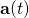is written as a truncated Fourier series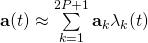,

where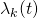is a convenience notation for either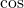or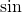. This sum approximation is then substituted into the governing equation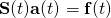,

and the unknown coefficients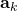are then solved with the Galerkin’s method.

This gives us a big block matrix equation, with the matrix blocks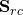equal to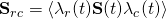,

where the angle brackets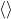correspond to calculating the time-average. From this matrix system, the coefficientscan then be solved.

### Implementation

However, this leaves us with the not-so-trivial problem of actually computing these blocks. As I described earlier, the stiffness matrix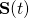can be divided into constant and time-dependent parts, i.e.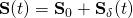.

Obviously, the static component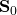is no problem to handle. However, this cannot be said for the time-dependent component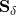.

Indeed, the most typical approach to model the rotation of the machine is to change the finite element mesh in the air-gap, where the stator and rotor are moving past each other. In this approach (and most others, for that matter), the entries of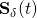will experience sharp, discontinuous changes as the elements of the mesh change. Thus, calculating the time-integral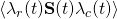accurately could be quite complicated indeed.

However, and luckily, this is a problem I have already solved. Remember earlier when I spoke about calculating the Fourier series of the matrix? In other words, I already know how to writeas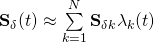,

and how to compute the coefficients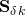.

### Number of required terms

Of course, having to compute a huge number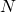of the terms would not be very practical, would it. I mean, that would be required to present the highly discontinuous entries ofaccurately, right?

Well, yes and no. Accurate representation ofwould indeed need quite a many terms. However, we are not interested in an accurate series approximation of– we are interested in calculating the matrix blocks!

Thus, let’s now substitute the approximation forhere, to get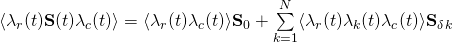.

In other words, we now longer have a time-average of a matrix – we have the time average of the product of three trigonometric functions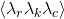. And this can be expressed in closed form quite easily.

And even more better, there are only a limited number of terms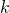for which the expression is non-zero. And these values ofcan also be determined analytically. Thus, it is usually sufficient to compute something like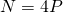of the air-gap matrix Fourier coefficients.

And this is obviously a much easier task to computing a total of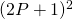of the matrix block integrals, like we would have been forced to do before.

Need help with electric motor design or design software? Let's get in touch - satisfaction guaranteed!Making time-stepping FEM faster, part 4

### 3 thoughts on “Making time-stepping FEM faster, part 4”

•Pavel Ponomarev says:

Hi,

Great posts on Electrical Engineering! Those information bombs really help with understanding of those FEM tricks. Thank you! And please write more=)

BR, Pavel

1.Antti (admin) says:

Hi Pavel,

Thank you so much for your words! Nice that you like them 🙂

And I will definitely continue writing 🙂

-Antti

•Antti (admin) says:

And in general: if you have questions or ideas for blog topics, I’m always open for them 🙂